# Bond Order Length and Energy Bond Order The

• Slides: 10Bond Order, Length, and Energy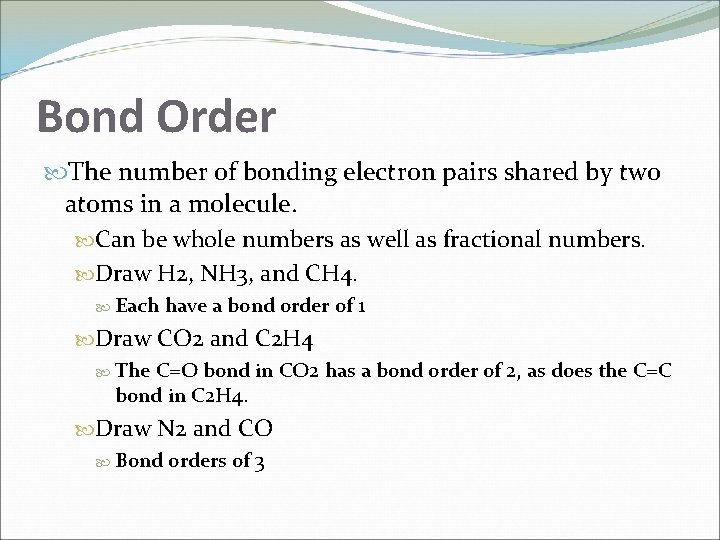Bond Order The number of bonding electron pairs shared by two atoms in a molecule. Can be whole numbers as well as fractional numbers. Draw H 2, NH 3, and CH 4. Each have a bond order of 1 Draw CO 2 and C 2 H 4 The C=O bond in CO 2 has a bond order of 2, as does the C=C bond in C 2 H 4. Draw N 2 and CO Bond orders of 3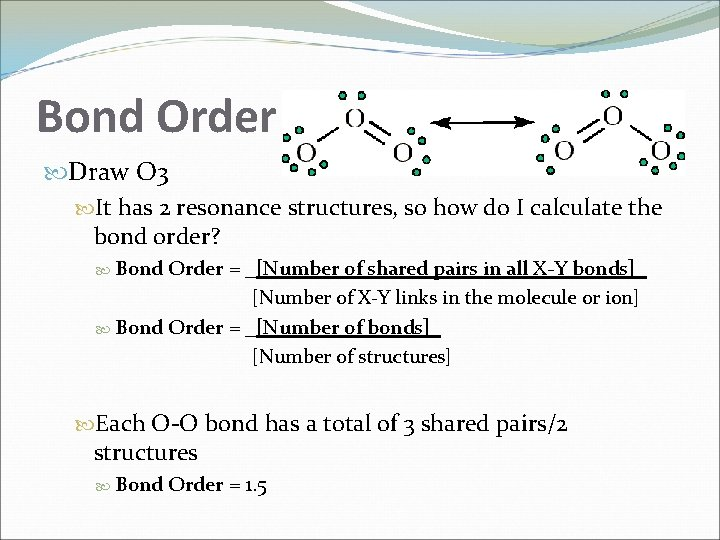Bond Order Draw O 3 It has 2 resonance structures, so how do I calculate the bond order? Bond Order = _[Number of shared pairs in all X-Y bonds]_ [Number of X-Y links in the molecule or ion] Bond Order = _[Number of bonds]_ [Number of structures] Each O-O bond has a total of 3 shared pairs/2 structures Bond Order = 1. 5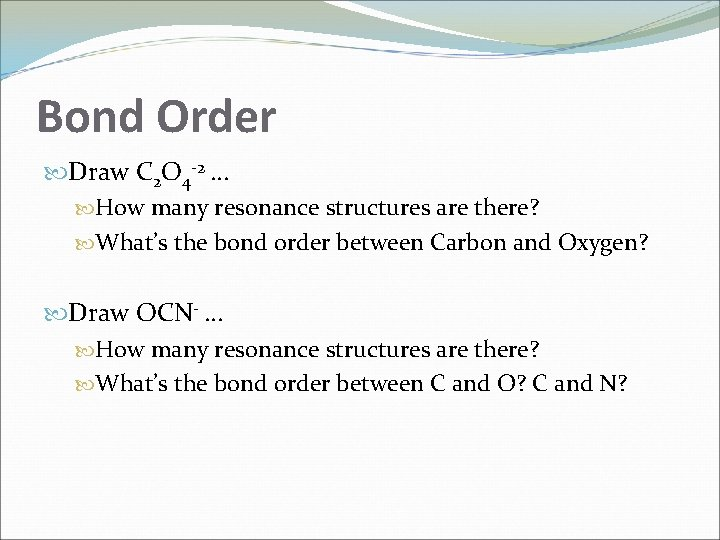Bond Order Draw C 2 O 4 -2 … How many resonance structures are there? What’s the bond order between Carbon and Oxygen? Draw OCN- … How many resonance structures are there? What’s the bond order between C and O? C and N?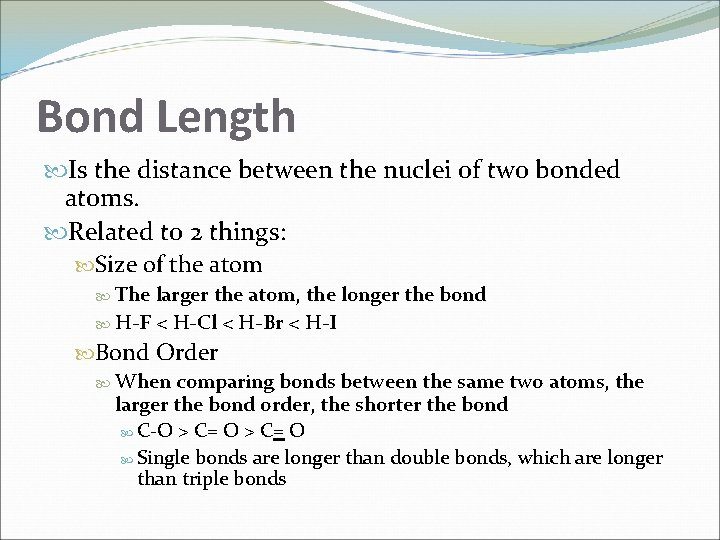Bond Length Is the distance between the nuclei of two bonded atoms. Related to 2 things: Size of the atom The larger the atom, the longer the bond H-F < H-Cl < H-Br < H-I Bond Order When comparing bonds between the same two atoms, the larger the bond order, the shorter the bond C-O > C= O Single bonds are longer than double bonds, which are longer than triple bonds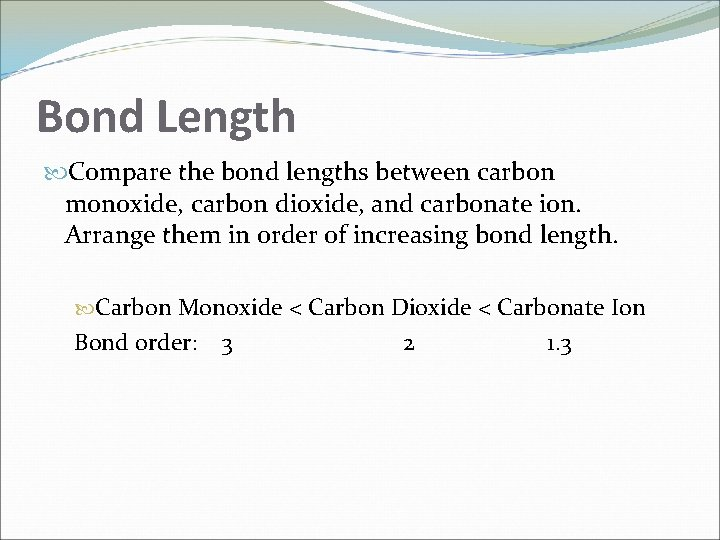Bond Length Compare the bond lengths between carbon monoxide, carbon dioxide, and carbonate ion. Arrange them in order of increasing bond length. Carbon Monoxide < Carbon Dioxide < Carbonate Ion Bond order: 3 2 1. 3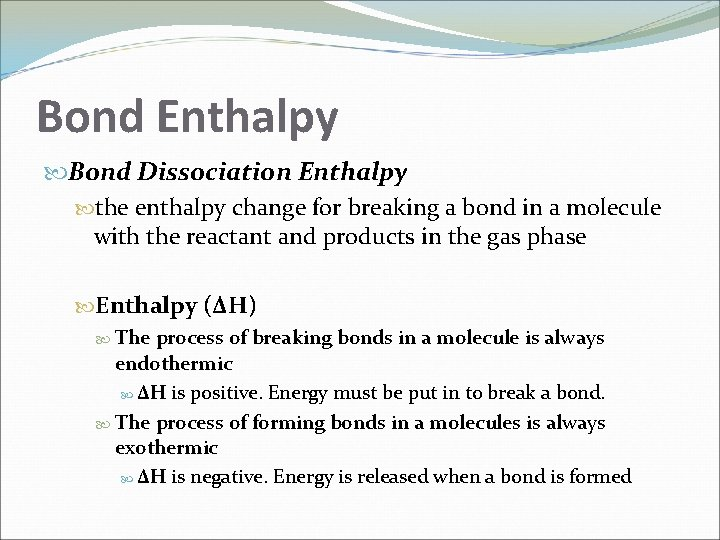Bond Enthalpy Bond Dissociation Enthalpy the enthalpy change for breaking a bond in a molecule with the reactant and products in the gas phase Enthalpy (ΔH) The process of breaking bonds in a molecule is always endothermic ΔH is positive. Energy must be put in to break a bond. The process of forming bonds in a molecules is always exothermic ΔH is negative. Energy is released when a bond is formed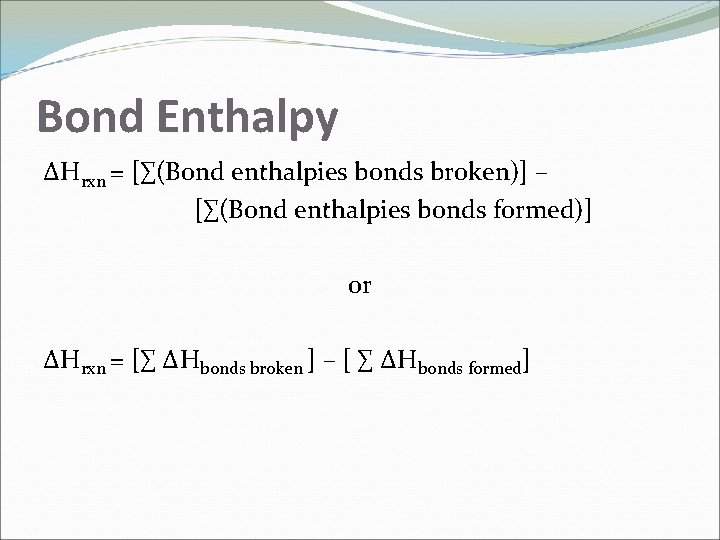Bond Enthalpy ΔHrxn = [∑(Bond enthalpies bonds broken)] – [∑(Bond enthalpies bonds formed)] or ΔHrxn = [∑ ΔHbonds broken ] – [ ∑ ΔHbonds formed]Practice Problems Compare the nitrogen-nitrogen bond in hydrazine, H 2 NNH 2, with that in “laughing gas”, N 2 O. In which molecule is the nitrogen-nitrogen bond shorter? In which is the bond stronger?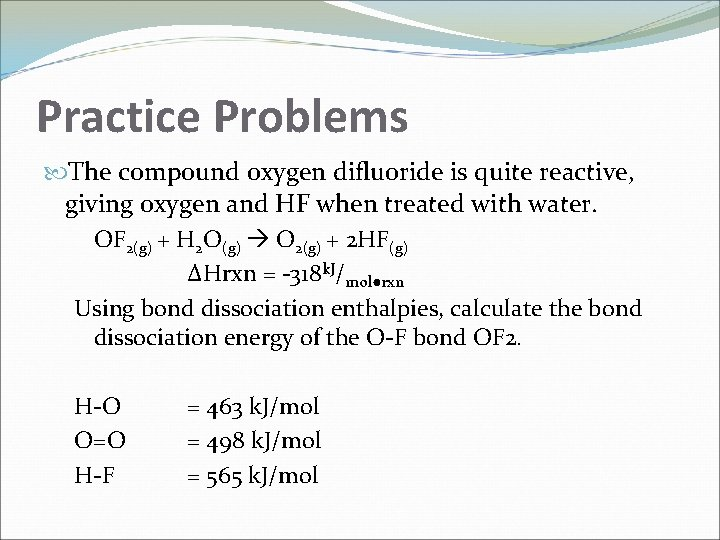Practice Problems The compound oxygen difluoride is quite reactive, giving oxygen and HF when treated with water. OF 2(g) + H 2 O(g) O 2(g) + 2 HF(g) ΔHrxn = -318 k. J/mol●rxn Using bond dissociation enthalpies, calculate the bond dissociation energy of the O-F bond OF 2. H-O O=O H-F = 463 k. J/mol = 498 k. J/mol = 565 k. J/mol